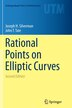Rational Points On Elliptic Curves

byJoseph H. Silverman, John T. Tate

Pricing and Purchase Info

\$75.63 online
\$85.50 list price save 11%
Earn 378 plum® points

Prices and offers may vary in store

Quantity:

In stock online

Ships free on orders over \$25

Not available in stores

The theory of elliptic curves involves a pleasing blend of algebra, geometry, analysis, and number theory. This volume stresses this interplay as it develops the basic theory, thereby providing an opportunity for advanced undergraduates to appreciate the unity of modern mathematics. At the same time, every effort has been made to use only methods and results commonly included in the undergraduate curriculum. This accessibility, the informal writing style, and a wealth of exercises makeRational Points on Elliptic Curvesan ideal introduction for students at all levels who are interested in learning about Diophantine equations and arithmetic geometry.

Most concretely, an elliptic curve is the set of zeroes of a cubic polynomial in two variables. If the polynomial has rational coefficients, then one can ask for a description of those zeroes whose coordinates are either integers or rational numbers. It is this number theoretic question that is the main subject ofRational Points on Elliptic Curves. Topics covered include the geometry and group structure of elliptic curves, the Nagell-Lutz theorem describing points of finite order, the Mordell-Weil theorem on the finite generation of the group of rational points, the Thue-Siegel theorem on the finiteness of the set of integer points, theorems on counting points with coordinates in finite fields, Lenstra's elliptic curve factorization algorithm, and a discussion of complex multiplication and the Galois representations associated to torsion points. Additional topics new to the second edition include an introduction to elliptic curve cryptography and a brief discussion of the stunning proof of Fermat's Last Theorem by Wiles et al. via the use of elliptic curves.

Joseph H. Silverman is Professor of Mathematics at Brown University. He is the author of over 100 research articles and numerous books on elliptic curves, diophantine geometry, cryptography, and arithmetic dynamical systems.John T. Tate is Professor Emeritus of Mathematics at The University of Texas at Austin and at Harvard University....